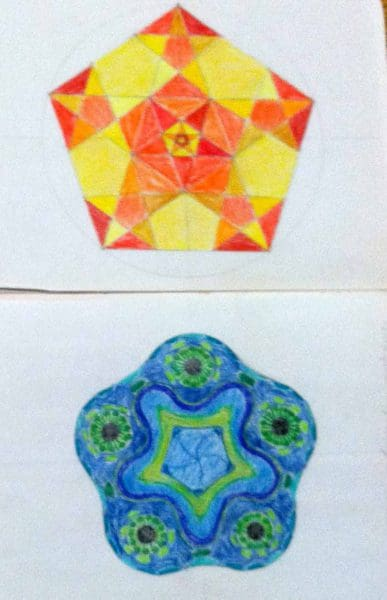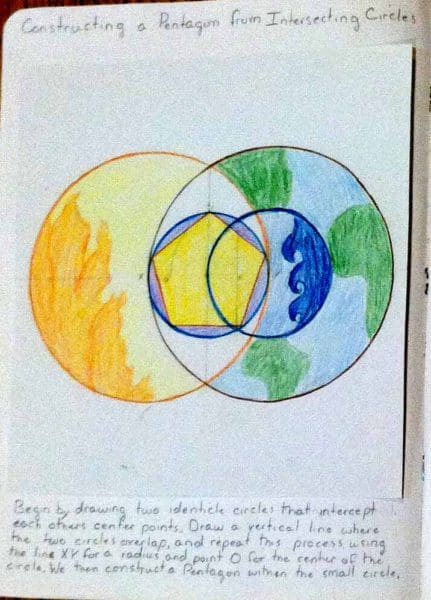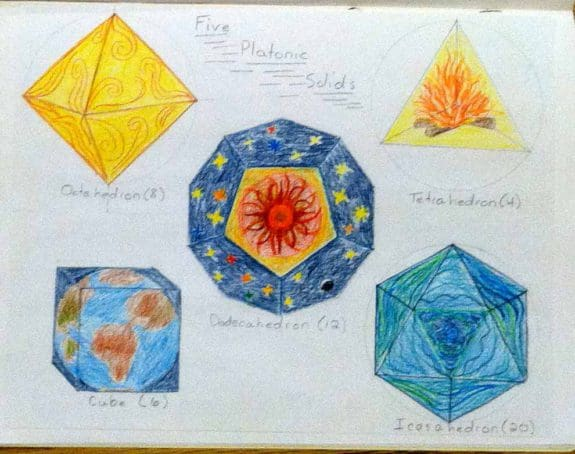# A Mathematics Main Lesson: Geometry in Man and Nature (7th and 8th)

By Barbara Benson

The  primary reference text recommended in A Rough Guide for Seventh Grade  is Mathematics Around Us. The primary text for Eighth Grade is Mathematics  in Nature, Space and Time. These are both great books with wonderful illustrations. I would like to share with you the 4 week main lesson outline that I put together for my children in eighth grade using these texts. I called it “Geometry in Man and Nature”. There are many topics in it that are primarily 7th grade material, either that we did not cover in 7th grade  or we used as a  review. The work on Platonic Solids is definitely an 8th grade topic. Additional sources that I used were the Modeling book for doing the  Platonic Solids from clay and  Ron Jarman’s Teaching Mathematics in Rudolf Steiner Schools for Classes I-VIII.Week One: History of Geometry; Proofs of Pythagorean Theorem; Spiral of Archimedes, Leaf Shapes

Days One and Two: Begin with a Geometry in history review which could even go back to look at the String, Straightedge and Shadow book used in earlier grades. Focus again on Pythagoras and the Pythagorean Theorem. I showed them a number of proofs for it and discussed them. She solved a navigation problem using the theorem:

“Suppose a ship near the equator sails 37 miles from P to Q; and it is found by observing the sun’s position and time on the clock that the ship is 35 miles further east than before. How far north has it sailed?” Answer: The area of the smallest square is 37 squared minus 35 squared ( 37squared = 1,369;  35 squared =1,225.   1,369- 1,225= 144 square miles or 12 squared , so R, the distance North is 12 miles. (This is from  Teaching Mathematics  )

Then she picked a proof that she liked, the Unfolded Version of Ibn Quarra’s Proof,  and drew the proof with colored squares and stated the theorem:” For a right angle triangle, the square on the hypotenuse is equal to the sum of the squares on the other two sides.”

Days Three and Four: We took a close look at the Spiral of Archimedes and my daughter did a compass drawing showing the spiral in points along the circles. Her text in the main lesson book stated:” Points are obtained by moving from radius to radius in equal steps towards the center. A sailor’s coiled ropes are a good example.”

Then we went on to explore and construct Leaf Shapes and drew these within the compass drawn circle. She put two of these leaf drawings into her main lesson book.

Week Two: Logarithmic Spiral, Shapes in Nature, Fibonacci Series

Days One and Two: We explored the Logarithmic Spiral and she did a full page color construction of it. Her text below it read: “By extending perpendicular lines from one radius to the next, we create a logarithmic spiral. This type of spiral rapidly whirls inwards, but never reaches the center of the circle.”

Days Three and Four: We looked at shell shapes that demonstrate the logarithmic spiral and she did a free hand drawing of a nautilus. Then she did a full page drawing of the nautilus showing how its proportions exhibit the Fibonacci series. Her text read:” Taking the ratio of two successive numbers in the series (1,1,2,3,5,8,13,21,34…) and then dividing each of the preceding numbers, we arrive at the following sequence of  numbers: 1/1=1; 2/1=2;  3/2= 1.5. 5/3=1.666 continuing until 2584/159=1.618033813 or phi.Finally we worked out the old rabbit problem using the series and then went to the piano to experience the keyboard. She drew out a portion of the keyboard showing the black keys ( 2 and then 3 for a total of 5) and the 8 white keys for a total of 13.

She concluded in her text: ” The Fibonacci sequence is  found in the piano, the nautilus shell, the seeds in a sunflower head and in the growth of certain trees as they branch off.”

Week Three: Golden Mean/Ratio/Rectangle

Days 1-4: This week we explored the Golden mean/ratio/rectangle. We carefully constructed a golden rectangle first and then a logarithmic spiral created by subtracting squares from a golden rectangle and then drawing quarter circles in each square to form the spiral. We saw the use of the golden proportion/ration in nature and art, exploring Leonardo’s Mona Lisa particularly. Then we worked to construct a pentagon from a golden rectangle and then finally the wonderful pentagram constructed within a pentagon within a circle. ( I definitely had to practice this one before class!) Here is some of her text beneath her drawings:

” The Golden Rectangle: Another geometrical form that is hidden in many works of art and creations of nature is the Golden Rectangle. We construct a Golden Rectangle by first drawing a square. The rectangle that is added to the square is itself a Golden Rectangle, and the sum of the square and the small rectangle is also a Golden Rectangle.”  ” A Logarithmic Spiral is created by subtracting squares from a Golden Rectangle and then drawing quarter circles in each square to form the spiral.”

“Constructing a Pentagram from a Golden Rectangle: Begin by constructing a Golden Rectangle and then using the line AB as a radius, draw two half circles from point A and C. The intersection created by the circles is labeled D. We then use the line AC as a radius and draw a line from A and C to create points E and F. Connect A to E and so on, until you have a pentagon.”

“Constructing a Pentagram within a Pentagon from a Circle: Begin by dividing a circle in half and label the line X, O (at the center) and Y and quarter the top and label it A. Divide the radius in half and label it P. Then , taking the distance of AP for a radius, we draw a section of a circle to intersect line X and label it Q. We then take the distance AQ for a radius which should go 5 times around the circle, thus creating a pentagon. It is now easy to make a Pentagram!”

Week Four: Continuing work with Pentagon/Pentagram; Platonic Solids

Days One and Two: Her first drawing was a colored  compass drawing on how to construct a Pentagon from Intersecting Circles. She decorated it to show the elements of earth, fire air and water. Her text read: ” Begin by drawing to identical circles that intercept each other’s center point. Draw a vertical line where the two circles overlap and repeat this process using the line XY for a radius and point O for the center of the circle. We then construct a pentagon within the small circle.”

She next did a colored complex pentagram construction and then a drawing of a marine animal showing pentagonal symmetry in the animal.

Days Three, Four, Five: Basic Geometric shapes; formula for Platonic Solids, Drawings of Five Platonic Solids: Her first page in this section included a drawing page of all the basic geometric shapes: square, rectangle, trapezium, rhombus, parallelogram, regular pentagon, regular hexagon, regular octagon, scalene triangle, isosceles triangle, circle, kite, equilateral triangle and oval (ellipse).

Next we worked with the Platonic Solids. After reviewing a little history of the solids and Plato’s writings on it, we went on to count the faces, edges and points of the solids. She had earlier in the year constructed the five solids out of cardstock. Now we looked at the Faces, Edges and Points (Vertices) of each of the shapes: Tetrahedron ( 4 faces, 6 edges and 4 points); Hexahedron ( 6 faces, 12 edges, 8 points); Octahedron ( 8 faces, 12 edges and 6 points); Dodecahedron (12 faces, 30 edges and 20 points) and Icosahedron (20 faces, 30 edges, and 12 points). She created a listing of this in her main lesson book and then wrote down the formula for the solids: ” F (faces) + P (points) = E (edges) +2.  So, 4+4 = 6+2 ; 6+8= 12 +2; 8+6= 12+2; 12+20= 30+2; 20 + 12= 30 +2.She then did a flat construction of all the five solids in the main lesson book, decorating them in color with Kepler’s earth, air, fire, water and cosmos designs. The Hexahedron or cube is earth; Octahedron is Air; Tetrahedron is Fire; Icosahedron is water; and Dodecahedron is the cosmos.

On the last day we did the fun and challenging metamorphosis of the five Platonic Solids using clay and carefully following the instructions in the Learning about the World Through Modeling book. It was an amazing exercise and I recommend it highly.

If you have time to add on a fifth week to your main lesson, it would be wonderful to cover the section on Rhythm and Cycles from Mathematics in Space and Time, especially focusing on the Earth’s elliptical path around the sun. We did not have time to do it in this lesson, although we worked on the concepts in Astronomy.

As you can see, there are many ways to approach the main lesson material from these books for seventh and eighth grade. Ours was just one approach, but it was one that heightened our reverence for how Creation geometrizes!

Happy Homeschooling,

Barbara

Christopherus Homeschool Consultant

Posted on July 21, 2016 in 7th Grade, 8th Grade, Barbara, Older Children, Waldorf Education